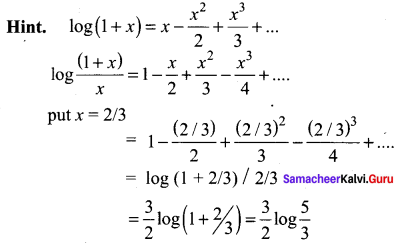## Tamilnadu Samacheer Kalvi 11th Maths Solutions Chapter 5 Binomial Theorem, Sequences and Series Ex 5.5

Choose the correct or the most suitable answer:

Question 1.
The value of 2 + 4 + 6 + … + 2n is …..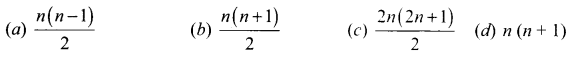Solution:
(d) n(n + 1)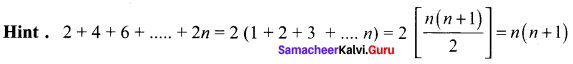Question 2.
The coefficient of x6 in (2 + 2x)10 is ……….
(a) 10C6
(b) 26
(c) 10C626
(d) 10C6210
Solution:
(d) 10C6210
Hint.
tr + 1 = 210(nCr)
To find coefficient of x6 put r = 6
∴ coefficient of x6 = 210 [10C6]

Question 3.
The coefficient of x8y12 in the expansion of (2x + 3y)20 is …….
(a) 0
(b) 28312
(c) 28312 + 21238
(d) 20C828312
Solution:
(d) 20C828312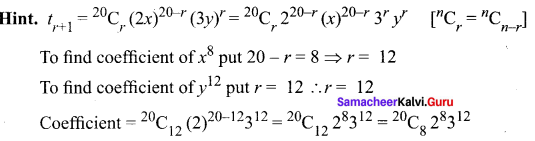Question 4.
If nC10 > nCr for all possible r, then a value of n is ……..
(a) 10
(b) 21
(c) 19
(d) 20
Solution:
(d) 20
Hint.
Out of 10C10, 21C10, 19C10 and 20C10, 20C10 is larger.

Question 5.
If a is the arithmetic mean and g is the geometric mean of two numbers, then ……..
(a) a ≤ g
(b) a ≥ g
(c) a = g
(d) a > g
Solution:
(b) a ≥ g
Hint. AM ≥ GM
∴ a ≥ g

Question 6.
If (1 + x2)2 (1 + x)n = a0 + a1x + a2x2 + …. + xn + 4 and if a0, a1, a2 are in AP, then n is ……
(a) 1
(b) 2
(c) 3
(d) 4
Solution:
(b or c)n = 2 or 3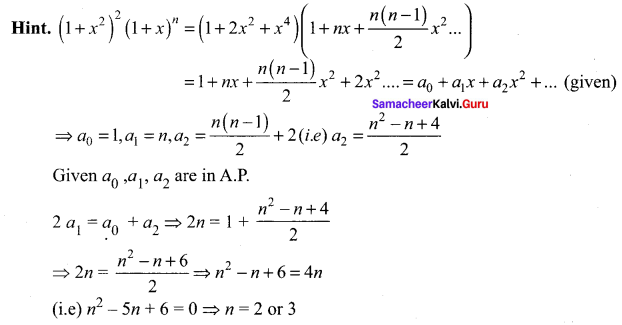Question 7.
If a, 8, b are in A.P, a, 4, b are in G.P, if a, x, b are in HP then x is ……
(a) 2
(b) 1
(c) 4
(d) 16
Solution:
(a) 2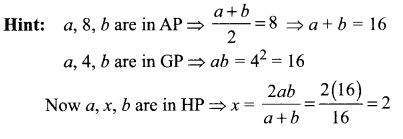Question 8.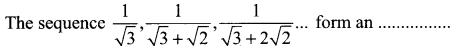(a) AP
(b) GP
(c) HP
(d) AGP
Solution:
(c) HPQuestion 9.
The HM of two positive numbers whose AM and GM are 16, 8 respectively is ………
(a) 10
(b) 6
(c) 5
(d) 4
Solution:
(d) 4
Hint.
Let the two numbers be a and b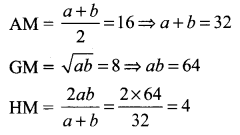Question 10.
If Sn denotes the sum of n terms of an AP whose common difference is d, the value of $$\mathrm{S}_{n}-2 \mathrm{S}_{n-1}+S_{n-2}$$ is ……
(a) d
(b) 2d
(c) 4d
(d) d2
Solution:
(a) d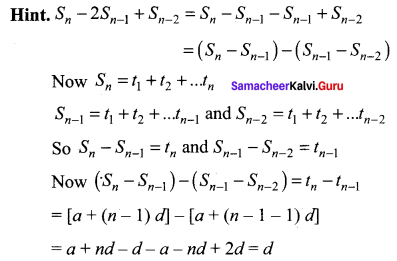Question 11.
The remainder when 3815 is divided by 13 is …….
(a) 12
(b) 1
(c) 11
(d) 5
Solution:
(a) 12
Hint.
3815 = (39 – 1)15 = 3915 – 15C1 3914(1) + 15C2 (39)13(1)2 – 15C3 (39)12(1)3 ….. + 15C14 (39)1(1) – 15C15(1)
Except -1 all other terms are divisible by 13.
∴ When 1 is added to it the number is divisible by 13. So the remainder is 13 – 1 = 12.

Question 12.
The nth term of the sequence 1, 2, 4, 7, 11, …… is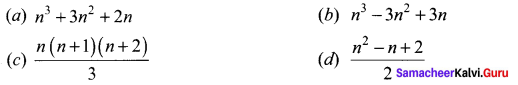Solution:
(d) $$\frac{n^{2}-n+2}{2}$$

Question 13.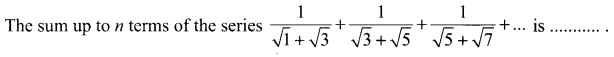Solution: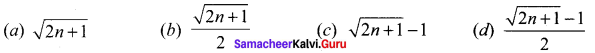Solution:
(d) $$\frac{\sqrt{2 n+1}-1}{2}$$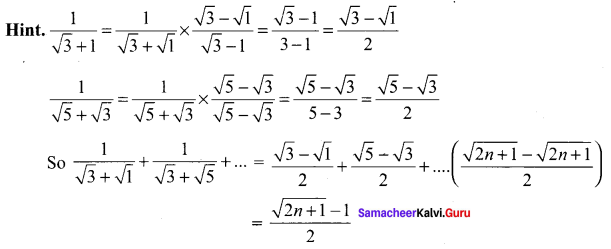Question 14.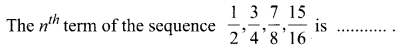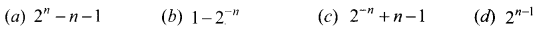Solution: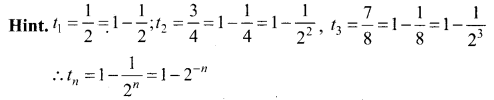Question 15.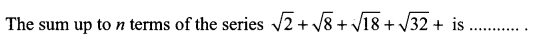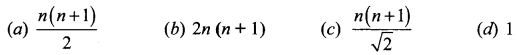Solution:
(c) $$\frac{n(n+1)}{\sqrt{2}}$$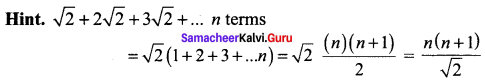Question 16.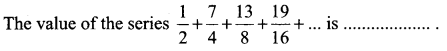(a) 14
(b) 7
(c) 4
(d) 6
Solution:
(a) 14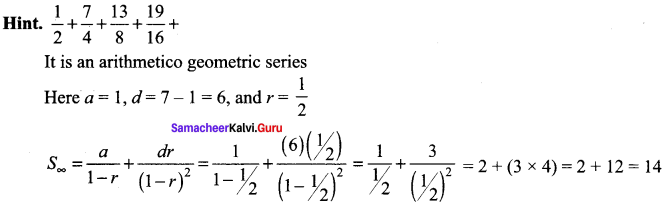Question 17.
The sum of an infinite GP is 18. If the first term is 6, the common ratio is ………
(a) $$\frac{1}{3}$$
(b) $$\frac{2}{3}$$
(c) $$\frac{1}{6}$$
(d) $$\frac{3}{4}$$
Solution:
(b) $$\frac{2}{3}$$
Hint: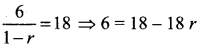18r = 18 – 6 = 12
r = 12/18 = 2/3

Question 18.
The coefficient of x5 in the series e-2x is ………
(a) $$\frac{2}{3}$$
(b) $$\frac{3}{2}$$
(c) $$\frac{-4}{15}$$
(d) $$\frac{4}{15}$$
(c) $$\frac{-4}{15}$$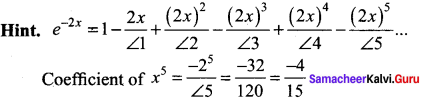Question 19.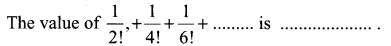(c) $$\frac{(e-1)^{2}}{2 e}$$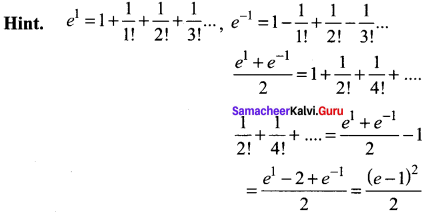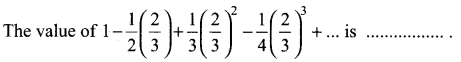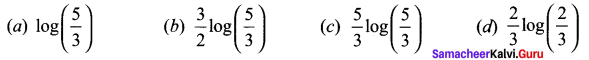(b) $$\frac{3}{2} \log \left(\frac{5}{3}\right)$$